Technical Article

# Understanding the Theory Behind Transient Thermal Impedance

March 16, 2023 by Christophe Vaucourt

## Transient thermal impedance is a measure of how a device behaves when pulsed power is applied. Transient thermal impedance is an important parameter, as it determines how the device behaves under low-duty cycles and low-frequency pulsed loads.

IC packages have many thermal metrics, such as θJA and ΨJT. These parameters make it simple to estimate junction temperature in a steady state. This article discusses thermal transient behaviors and presents the basic theory regarding thermal impedance.

### Thermal Parameters Overview

The thermal properties of flip-chip IC packages are characterized by their θJA, ΨJT, and ΨJB parameters. θJA is the junction-to-ambient thermal resistance (in °C/W), which is a system-level parameter that significantly depends on system properties, such as the design and layout of the PCB on which the part is mounted. The board acts as a heatsink that is soldered to the leads of the device. For natural convection heat transfer, more than 90% of the heat is dissipated by the board and not from the surfaces of the package. θJA can be calculated with Equation (1):

$\theta_{JA}-\frac{T_{J}-T_{A}}{P_{D}}$ (1)

Where TJ is the junction temperature (in °C), TA is the ambient temperature (in °C), and PD is the device’s heat dissipation (in W).

ΨJT is the characterization parameter that measures the temperature change between TJ and the temperature on the top of the package (in °C/W). Because the heat flowing from the die to the top of the package is unknown, ΨJT is not the true junction-to-top thermal resistance, but it is assumed by the circuit designer to be the total power of the device. Although this assumption is invalid, ΨJT is still a useful parameter since its characteristics are similar to that of the IC package’s application environment. For example, thinner packages have smaller ΨJT values.

Note that ΨJT varies slightly due to both board construction and airflow conditions. ΨJT can be estimated with Equation (2):

$\Psi_{JT}-\frac{T_{J}-T_{C}}{P_{D}}$ (2)

ΨJB can allow system designers to calculate the device’s junction temperature based on a board’s measured temperature. The ΨJB matric should be close to θJB, as the PCB dissipates most of the heat of the device. TJ can be calculated with Equation (3):

$T_{J}=T_{PCB}+(\Psi_{JB}XP_{D})$ (3)

Where TPCB is the board’s temperature close to the package’s exposed pad (in °C).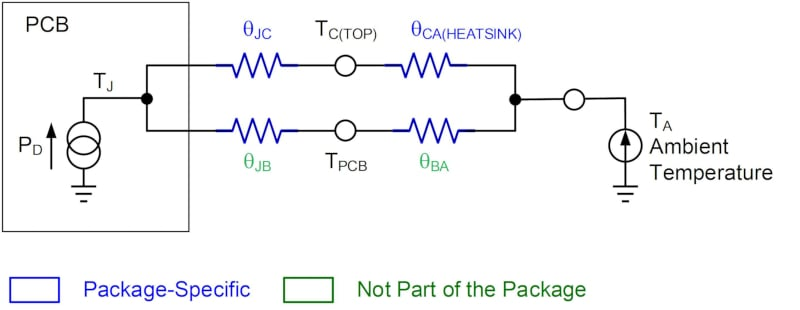##### Figure 1. Junction-to-Ambient Thermal Resistance. Image used courtesy of Bodo’s Power Systems [PDF]

Figure 1 shows a diagram that explains the junction-to-ambient thermal resistance.

A lower θJA is mainly achieved by reducing the resistance from the PCB’s thermal plane. In applications where conduction is the primary method of heat transfer (meaning that convection cooling is restricted), the PCB’s power plane area has the most significant effect on θBA

### Thermal Properties

In applications such as motor drivers, the high-power pulse width is limited to a few tens or hundreds of milliseconds, which means designers must consider the effects of thermal capacitance. If the thermal capacitance is sufficiently large, it can limit the junction temperature to remain within the device’s ratings, even in the presence of high dissipation peaks. Proper thermal management improves the device’s performance and reliability.

There are three mechanisms by which heat can be transferred: conduction, convection, and radiation.

### Conduction

Conduction is important because it is the surface area that eventually dissipates the heat. Through conduction, heat spreads out the required surface area. Heat transfer through conduction is governed by Fourier’s law, which states that the rate of heat flow through a material is directly proportional to the material’s cross-sectional area and the temperature difference across the material; conversely, the heat flow is inversely proportional to the material’s thickness. Some materials (e.g. copper) conduct heat more effectively than others (e.g. FR4). Table 1 shows the thermal conductivity factor (K) for different materials. These common materials have significantly different thermal conductivity factors.

##### Table 1. Material Thermal Conductivity
 Materials Conductivity (W/m.K) Air 0.025 FR4 PCB dielectric 0.35 Mold compound 1 Solder 62 Silicon(die) 148 Aluminum 247 Copper 398

### Convection

Convection is the method of moving heat from a material’s surface to the air. The temperature rise is a function of the power dissipated, and it is inversely proportional to the surface area and the heat transfer coefficient (h). h is a function of the air speed and the temperature difference between the board and the ambient air.

Thermal radiation involves the transfer of heat via electromagnetic waves. The rate of heat flow is directly proportional to the surface area and to the temperature of the radiating element (e.g. board, component) to the fourth power.

Heat transfer via conduction is most applicable to semiconductors in high-power applications. The standard description of the IC package’s thermal performance, θJA, is of little help in pulsed applications and leads to a redundant and prohibitive thermal design.

Instead, the complete thermal impedance of a device can be modeled by combining two elements: thermal resistance and thermal capacitance.

Thermal capacitance (CTH) is a measure of a component’s ability to accumulate heat, similar to how a capacitor accumulates a charge. For a given structural element, CTH depends on the specific heat (c), volume (V), and density (d). CTH (in J/°C) can be estimated with Equation (4):

$\tau = \theta\times C$ (4)

The electrical analogy for a given application’s thermal behavior (consisting of an active device, package, PCB, and external ambient) is a chain of RC cells, each having a characteristic time constant (τ). τ can be calculated with Equation (5):

$t_{P}\xrightarrow{\text{lim}}\infty\,Z_{TH(JA)}=\theta_{JA}$ (5)

Figure 2 shows how each cell contributes to the transient thermal impedance of a packaged device using a simplified electrical model.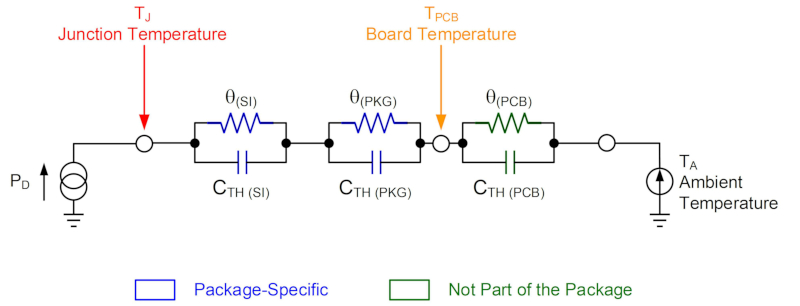### Pulse Power Operation

When a power device is subjected to a pulsed load, it can support higher peak power dissipation. Power packages have a definite thermal capacity, which means that the critical TJ is not reached immediately, even when excessive power is being dissipated in the device. The power dissipation limit may be extended for intermittent operation. The time length of the extension depends on the duration of the operation period (also known as the pulse duration) and the frequency during which the operation occurs (also known as the duty factor).

If power is applied to a device, the die immediately starts to warm up (see Figure 3).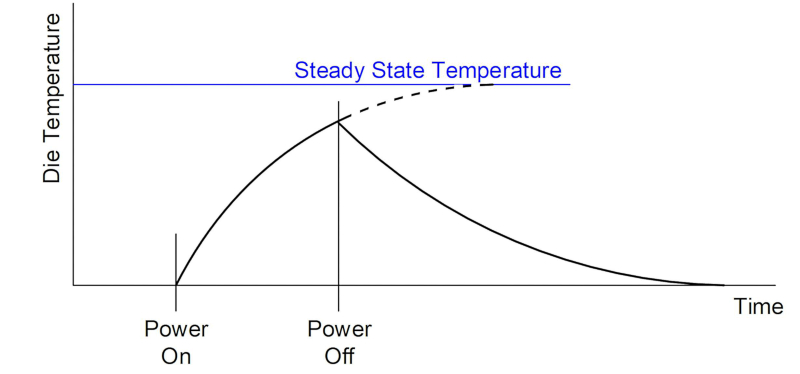##### Figure 3. Die Heating/Cooling: Single Pulse. Image used courtesy of Bodo’s Power Systems [PDF]

If the power continues dissipating, a balance is struck between heat generation and heat removal, which stabilizes TJ. Some heat energy is stored by the device’s thermal capacity. The stable conditions are determined by the thermal resistances, which are associated with the transistor and its thermal environment.

When power stops dissipating, the device cools down, and the heating and cooling laws are identical (see Figure 3). However, if power dissipation stops before the transistor’s temperature stabilizes, then the peak values of TJ are below the values that are reached for the same level of continuous power dissipation (see Figure 3).

If the second pulse is identical to the first, then the peak temperature attained by the device at the end of the second pulse is greater than the peak temperature at the end of the first pulse. Additional pulses build up until the temperature reaches a new, stable value (see Figure 4). Under these stable conditions, the device’s temperature fluctuates above and below the mean.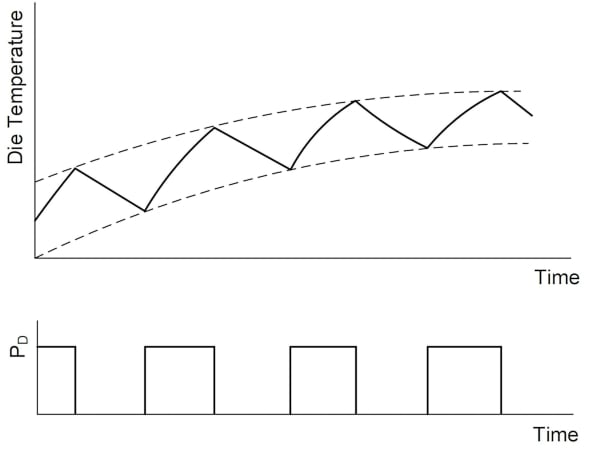##### Figure 4. Die Heating/Cooling: Repetitive Pulses. Image used courtesy of Bodo’s Power Systems [PDF]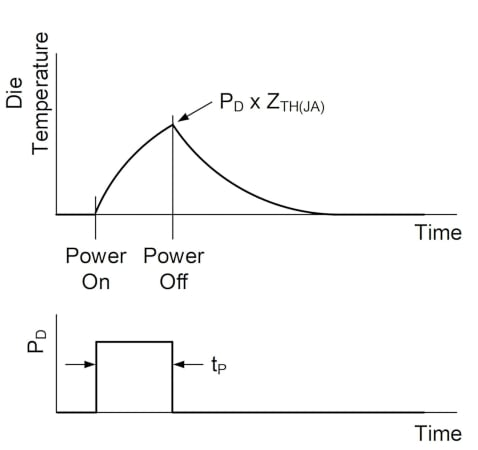##### Figure 5. Short Single Power Pulse. Image used courtesy of Bodo’s Power Systems [PDF]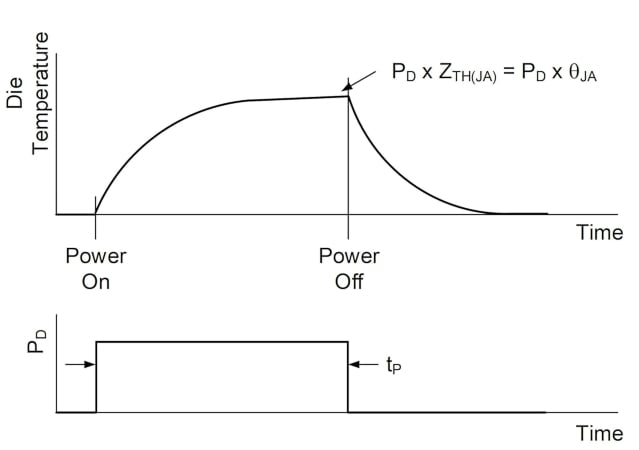##### Figure 6. Long Single Power Pulse. Image used courtesy of Bodo’s Power Systems [PDF]

If the junction temperature following a series of pulses becomes excessively high (e.g. TJ > 125°C), then the device may experience reduced electrical performance and life expectancy. This can occur with high-power pulses with low-duty cycles, even when the average power is below the device’s DC rating. Figure 5 shows a short, single-power pulse.

As the pulse duration increases, TJ approaches a stationary value toward the end of a pulse (see Figure 6).

The thermal impedance (ZTH(JA)) reflects the temperature rise produced by time-limited power pulses. This thermal impedance offers a simple way to estimate the device’s junction temperature under transient power dissipation conditions.

The transient thermal impedance tends to become equal to the thermal resistance for continuous power dissipation, estimated with Equation (6):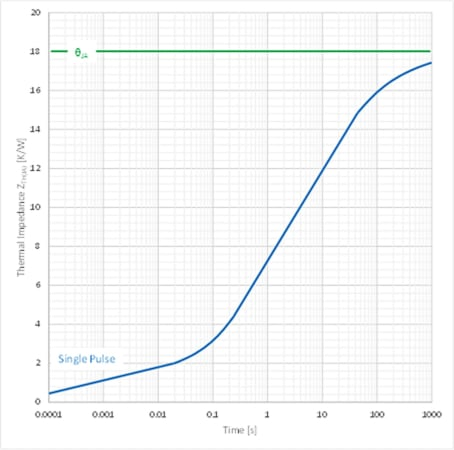##### Figure 7. Transient Impedance ZTH(JA) vs. Time. Image used courtesy of Bodo’s Power Systems [PDF]

As the repeat rate becomes smaller, the junction tends to cool down completely between pulses, so that each pulse can be treated individually.

For power packages, transient thermal effects die out within approximately 0.1 to 100 seconds. This time depends on the chip size, the package type, and size. In addition, it is greatly affected by the PCB stack-up and layout.

A PCB acts as a heatsink, providing path(s) for the IC package to effectively transfer heat to the board and the adjacent environment. Therefore, maximizing the area of the metal traces where the power and ground pin(s) of the package are located is important for effective heat transfer.

The package’s thermal performance is not greatly affected by TA and PD. Power pulses with excessive durations during this time have an effect that is similar to a continuous load.

### Effective Transient Thermal Impedance

The junction temperature influences many operational parameters, as well as the device’s operational lifetime. The most challenging aspect of designing a high-power circuit is determining whether a specific device can support the relevant application requirements.

The effective transient thermal impedance is influenced by many factors, including copper area and layout, heating from adjacent devices, the thermal mass of adjacent devices on the PCB, and airflow around the device. To accurately estimate the temperature rise, it is best to characterize the thermal impedance directly in the application circuit.

If you want to learn more, join Monolithic Power Systems´ webinar on March 22nd.